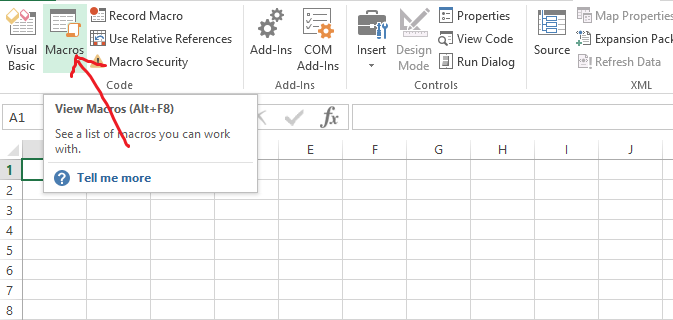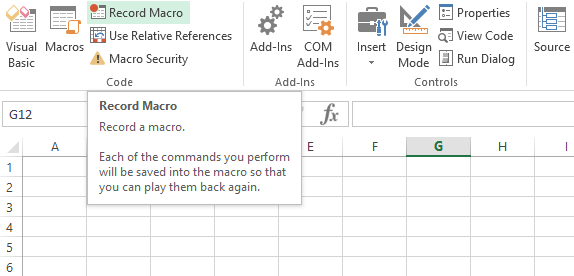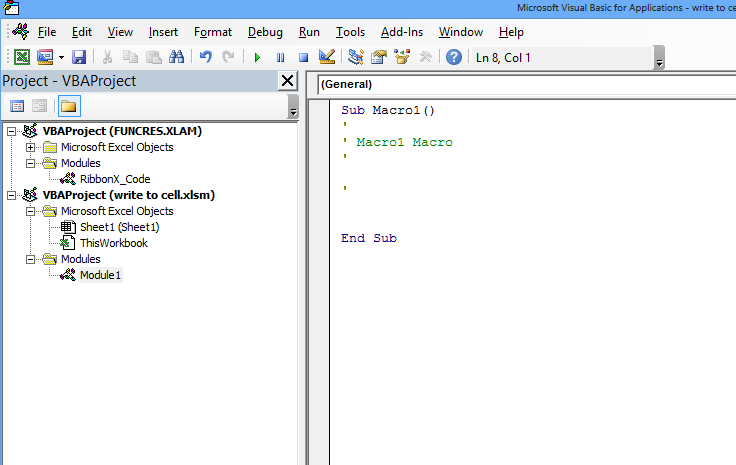### How To Loop Through Range In Excel

In this article we will learn how to use Excel macros to loop through a range with different techniques. Lets start with how to create an Excel module and Subroutine to write the code.

After opening the Excel file, click the developer tab.Ten go to Macros.If no macro is present we can click record macro and stop macro and then click macros:New window appears.Then click Edit. It will open the recorded macro as macro1 already created:Now under this module we can learn how to loop through a Range using macros.

Please copy this macro to the module 1:

```Sub Macro_loop_range()
Application.ScreenUpdating = False
Dim c As Range
For Each x In Range("A1:B10")
If x.Value = "Name" Then
MsgBox "Name found at " & x.Address
End If
Next c
End Sub```

In this Macro the range we are looping is “A1:B10” which can be changed as per the requirements.

X.value will loop through this range and it will check if the word “Name” exists anywhere and if found it will display the address of the cell. We can have other logic to search the loop as well.

Next I will show you a macro which will loop through a column:

```Sub Macro_loop_range2()
Application.ScreenUpdating = False
Dim c As Range
For Each x In Range("A:A")
If x.Value = "Name" Then
MsgBox "Name found at " & x.Address
End If
Next c
End Sub```

Here we are searching the entire column A and using the range as “A:A”.

Next I will show you a macro which will loop through a Row:

```Sub Macro_loop_range3()
Application.ScreenUpdating = False
Dim c As Range
For Each x In Range("1:1")
If x.Value = "Name" Then
MsgBox "Name found at " & x.Address
End If
Next c
End Sub```

Here we are searching the entire Row 1 and using the range as “1:1”.

## Template

```Further reading: